Examples

Chapter 6 Class 11 Permutations and Combinations
Serial order wise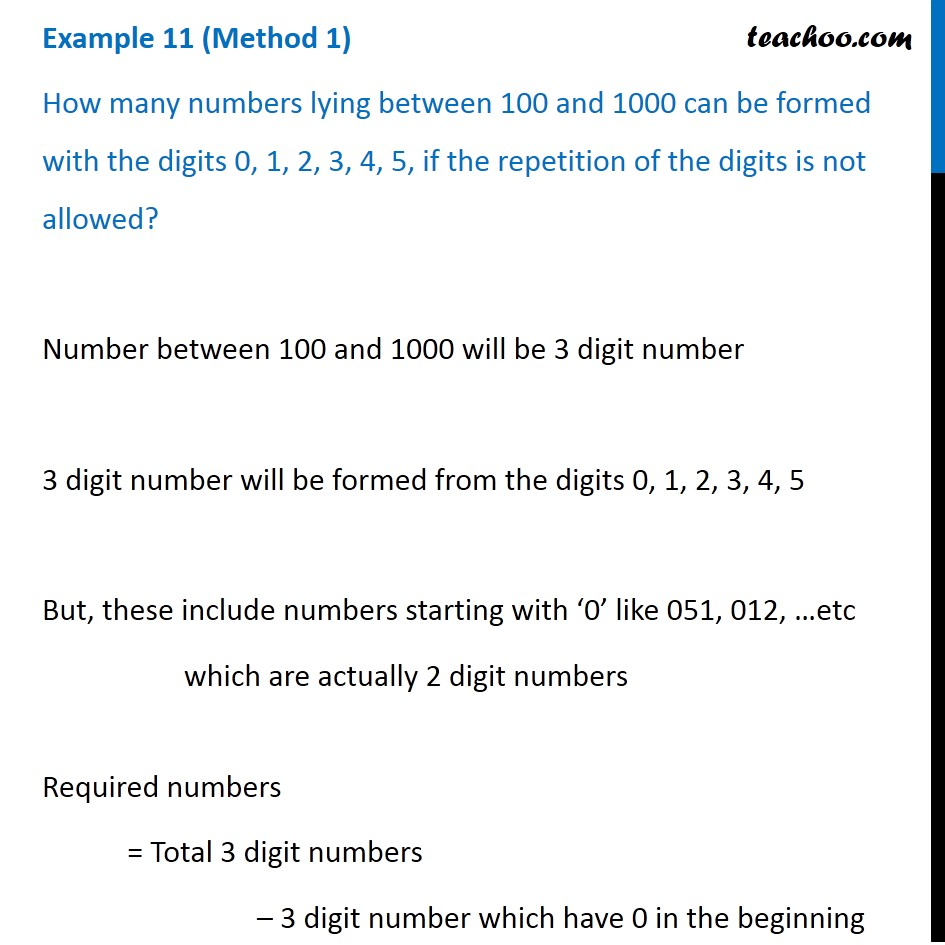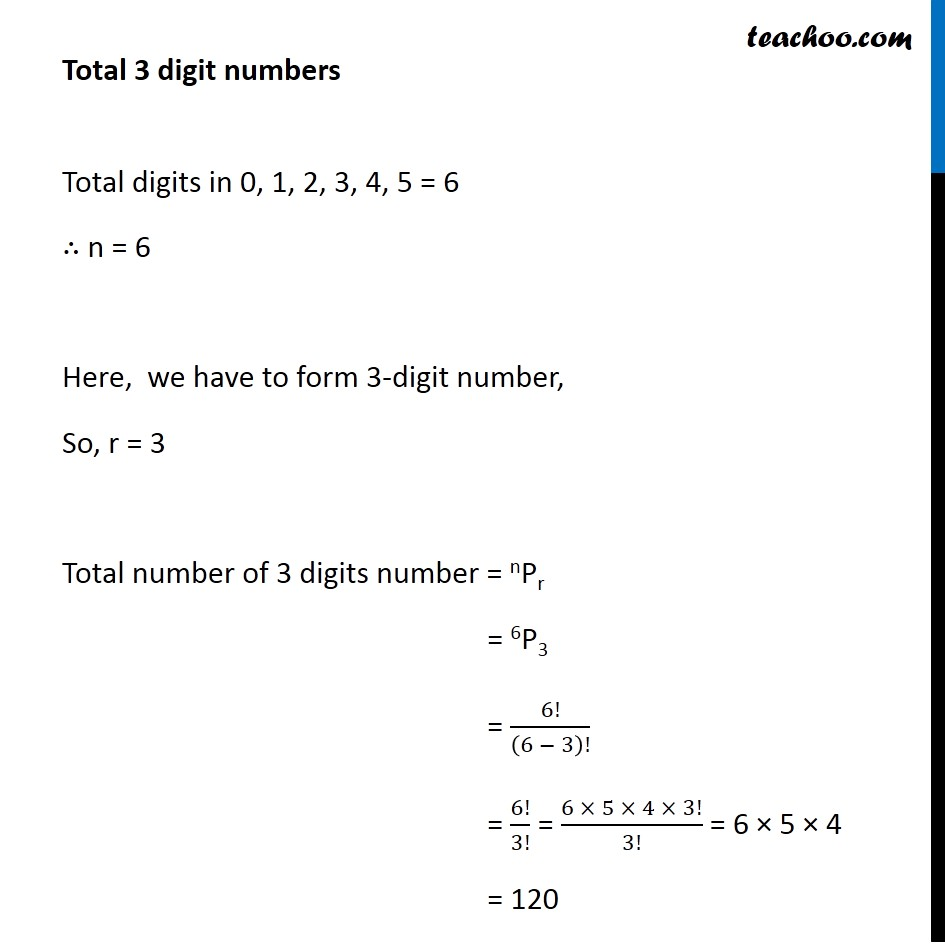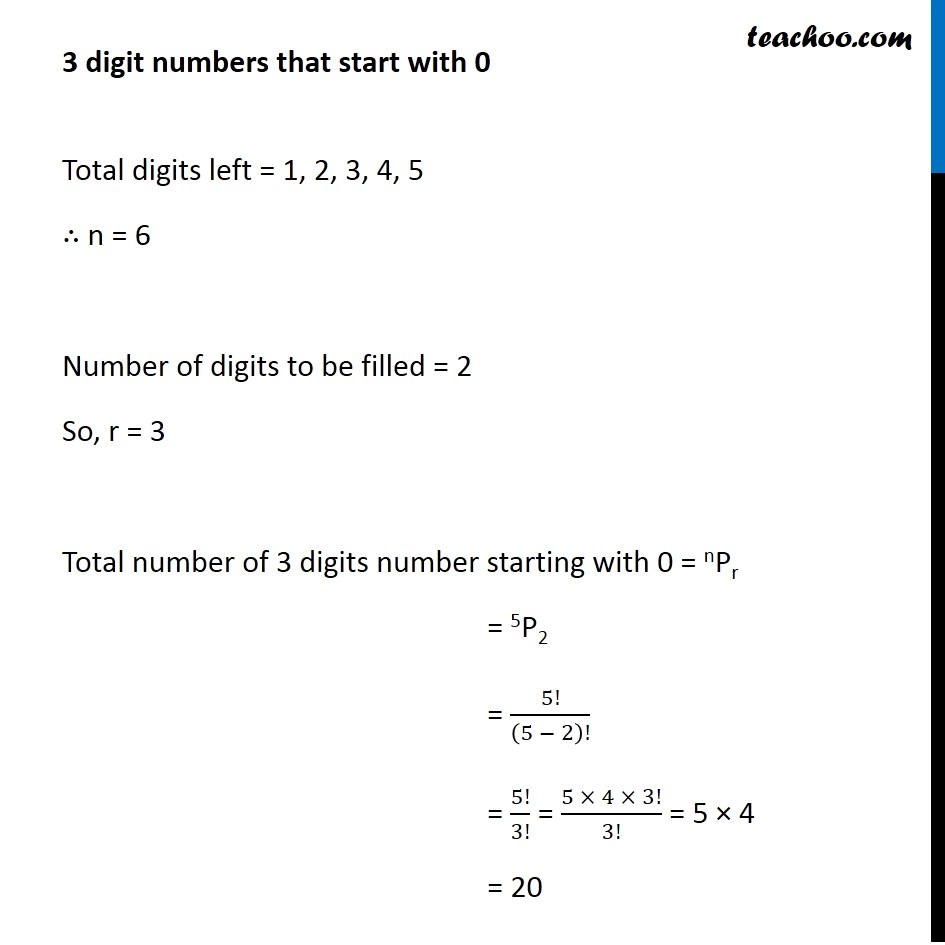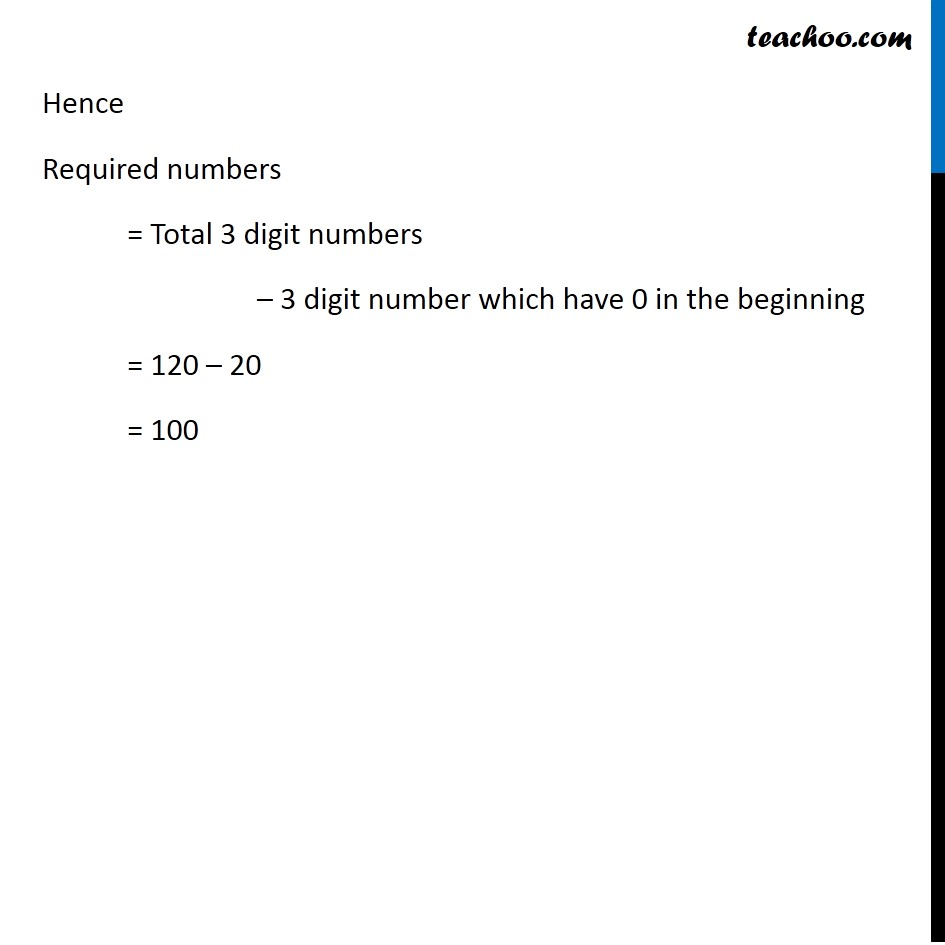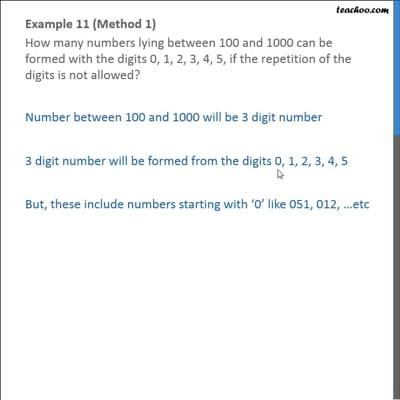This video is only available for Teachoo black users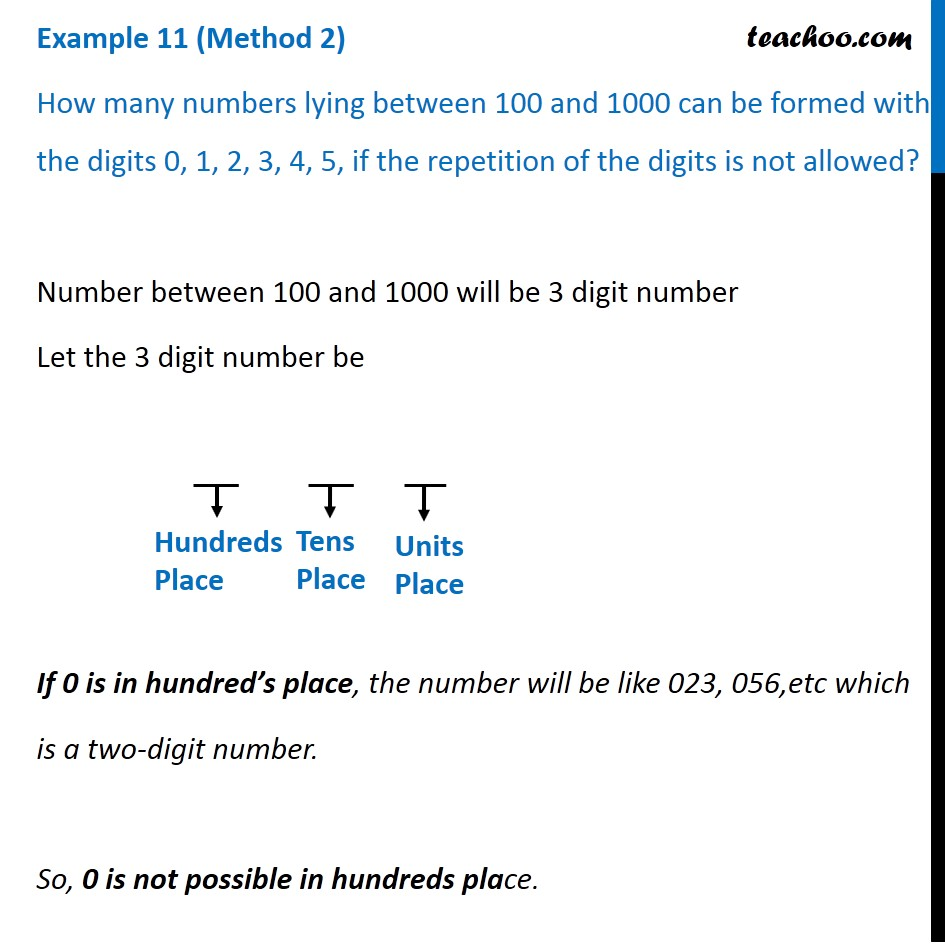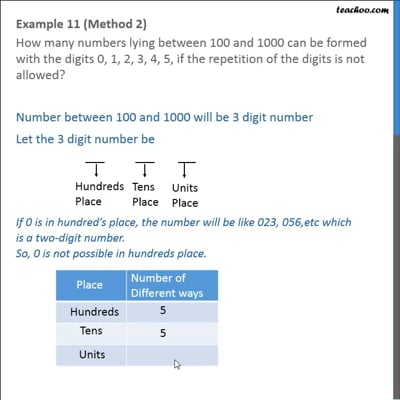This video is only available for Teachoo black users

Learn in your speed, with individual attention - Teachoo Maths 1-on-1 Class

### Transcript

Example 11 (Method 1) How many numbers lying between 100 and 1000 can be formed with the digits 0, 1, 2, 3, 4, 5, if the repetition of the digits is not allowed? Number between 100 and 1000 will be 3 digit number 3 digit number will be formed from the digits 0, 1, 2, 3, 4, 5 But, these include numbers starting with ‘0’ like 051, 012, …etc which are actually 2 digit numbers Required numbers = Total 3 digit numbers – 3 digit number which have 0 in the beginning Total 3 digit numbers Total digits in 0, 1, 2, 3, 4, 5 = 6 ∴ n = 6 Here, we have to form 3-digit number, So, r = 3 Total number of 3 digits number = nPr = 6P3 = 6!/(6 − 3)! = 6!/3! = (6 × 5 × 4 × 3!)/3! = 6 × 5 × 4 = 120 3 digit numbers that start with 0 Total digits left = 1, 2, 3, 4, 5 ∴ n = 6 Number of digits to be filled = 2 So, r = 3 Total number of 3 digits number starting with 0 = nPr = 5P2 = 5!/(5 − 2)! = 5!/3! = (5 × 4 × 3!)/3! = 5 × 4 = 20 Hence Required numbers = Total 3 digit numbers – 3 digit number which have 0 in the beginning = 120 – 20 = 100 Example 11 (Method 2) How many numbers lying between 100 and 1000 can be formed with the digits 0, 1, 2, 3, 4, 5, if the repetition of the digits is not allowed? Number between 100 and 1000 will be 3 digit number Let the 3 digit number be If 0 is in hundred’s place, the number will be like 023, 056,etc which is a two-digit number. So, 0 is not possible in hundreds place. Number of 3 digit numbers = 5 × 5 × 4 = 100